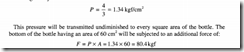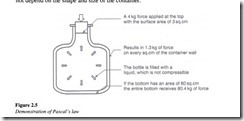# Pressure and flow:Pascal’s law.

### Pascal’s law

The underlying principle of how fluids transmit power is revealed by Pascal’s law. Pascal’s law states that the pressure applied to a confined fluid is transmitted undiminished in all directions. This law forms the basis for understanding the relationship between force, pressure and area, which can be mathematically expressed as:

Force = Pressure x Area or

Pressure =Force /Area

The transmitted pressure acts with equal force on every unit area of the containing vessel and in a direction at right angle to the surface of the vessel exposed to the liquid.

Pascal’s law can be illustrated by the following example.

A bottle is filled with a liquid, which is not compressible. A force of 4 kg is applied to the stopper whose surface area is 3 cm2• Let’s assume that the area of the bottle bottom is 60 cm2• If the stopper is inserted into the bottle mouth, with a force of 4 kg such that it

makes contact with the liquid, then the pressure exerted by the stopper on the liquid in the bottle is given by:This force could break most bottles. This shows why a glass bottle filled with liquid can break if the stopper is forced into its mouth.